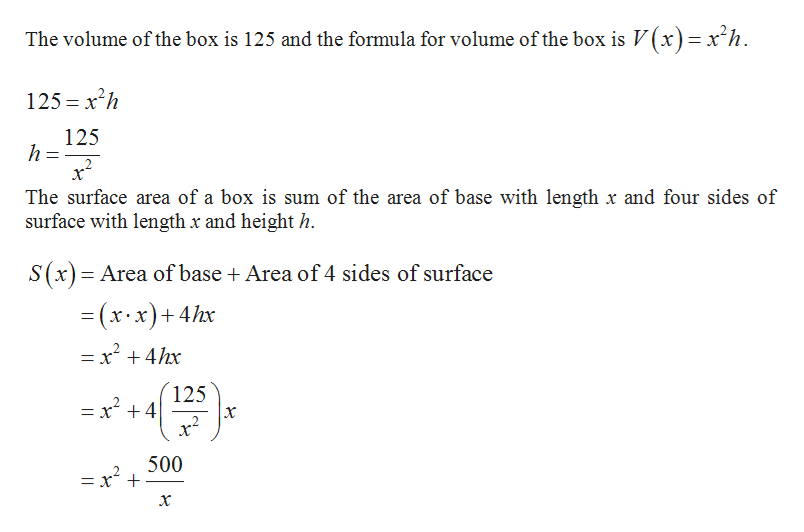Optimal boxes Imagine a lidless box with height h and a square base whose sides have length x. /the box must have a volume of 125 ft^3.Estimate the value of x that produces the box with a minimum surface area.The first part is to find and graph the function S(x), that gives the surface area of the box, for all values of x>0I just need to know how to find the minimum surface area.

Question

Optimal boxes Imagine a lidless box with height h and a square base whose sides have length x. /the box must have a volume of 125 ft^3.

Estimate the value of x that produces the box with a minimum surface area.

The first part is to find and graph the function S(x), that gives the surface area of the box, for all values of x>0

I just need to know how to find the minimum surface area.

Step 1

Let x be the length of the box and h be ...help_outlineImage TranscriptioncloseThe volume of the box is 125 and the formula for volume of the box is V(x)=x'h 125 xh 125 h The surface area of a box is sum of the area of base with length x and four sides of surface with length x and height h. S(x) Area of base Area of 4 sides of surface (x x)+4hx = x4hx 125 = x 4 2 500 = x t x fullscreen

Want to see the full answer?

See Solution

Want to see this answer and more?

Our solutions are written by experts, many with advanced degrees, and available 24/7

See Solution
Tagged in

Calculus